# 时间序列学习2：趋势

trend:模型随着移动平均值和time dummy的变化

1.什么是趋势？

2.移动平均线图（moving average plots）
sliding window

3.Engineering Trend

``````target = a*time +b
``````

fit many other kinds of trend through transformations of the time dummy.

``````target = a * time**2 + b* time + c
``````

3.例子：Tunnel Traffic

（1）让我们做一个移动平均线图，看看这个系列有什么样的趋势。由于这个系列有每日观察，让我们选择一个 365 天的窗口来平滑一年内的任何短期变化。要创建移动平均线，首先使用（rolling）滚动方法开始窗口计算。按照这个方法计算窗口的平均值。正如我们所看到的，隧道流量的趋势似乎是线性的。

• tunnel.rolling()

• tunnel.plot()
(2)在1中，我们直接在 Pandas 中设计了我们的时间虚拟机。然而，从现在开始，我们将使用 statsmodels 库中的一个名为DeterministicProcess 的函数。使用这个函数将帮助我们避免一些棘手的失败案例，这些案例可能会随着时间序列和线性回归而出现。 order 参数是指多项式顺序：1 表示线性，2 表示二次，3 表示三次，依此类推。

• DeterministicProcess

``````from statsmodel.tsa.deterministic import DeterministicProcess
``````

(3)预测模型

``````from sklearn.linear_model import LinearRegression
``````

``````X = dp.out_of_sample(steps = 30)
y_fore = pd.Series(model.predict(X),index = X.index)
``````

## 练习

1.制作一个移动平均线图来估计这个系列的趋势。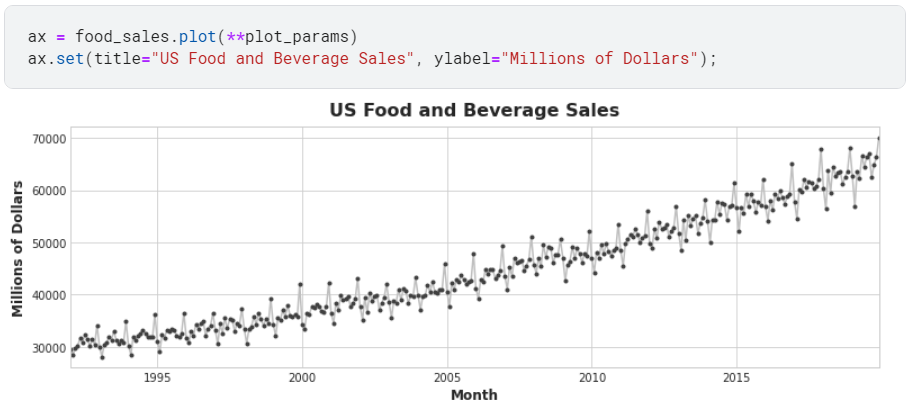``````#添加移动平均
trend = food_sales.rolling(window=12,center=True,min_periods=6,).mean()
#绘图
ax = food_sales.plot(**plot_params,alpha = 0.5)
ax = trend.plot(ax= ax,linewidth = 3)
``````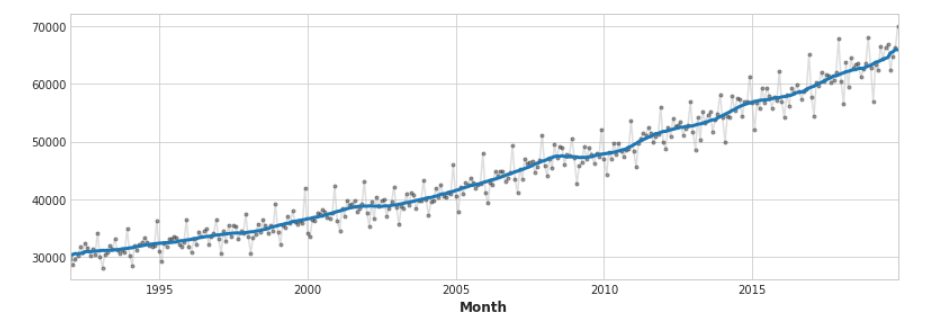2.辨别趋势（identify trend）

``````trend = average_sales.rolling(window=365,center=True,min_periods=183,).mean()
ax = average_sales.plot(**plot_params,alpha=0.5)
ax = trend.plot(ax=ax,linewidth = 3)
``````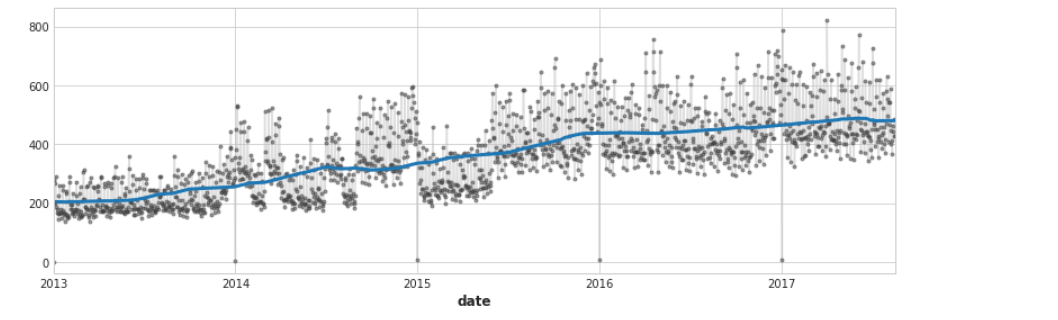3.创建趋势特征

``````from statsmodels.tsa.deterministic import DeterministicProcess
y = average_sales.copy()
#创建3次趋势模型
dp = DeterministicProcess(index=y.index,order=3)
X = dp.in_sample()
X_fore = dp.out_of_sample(steps =90 )

``````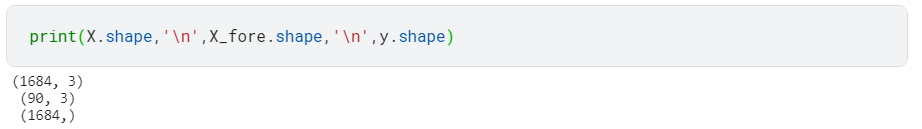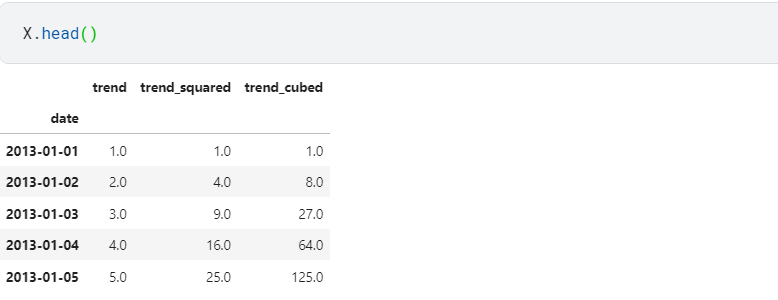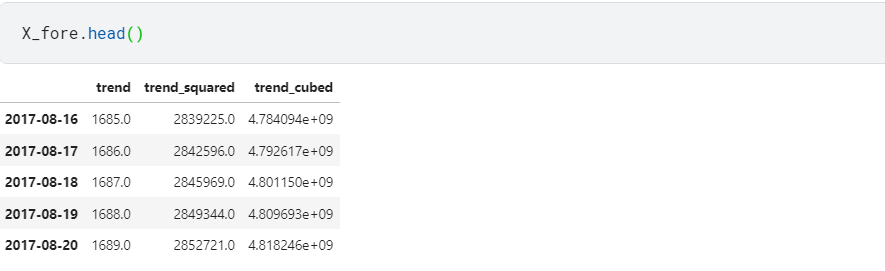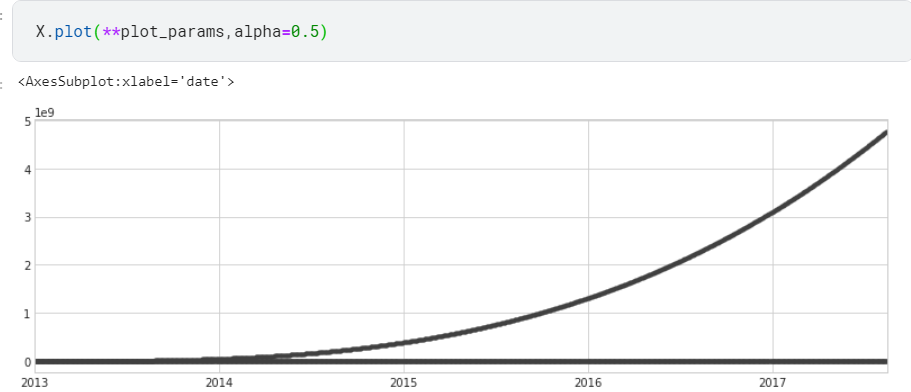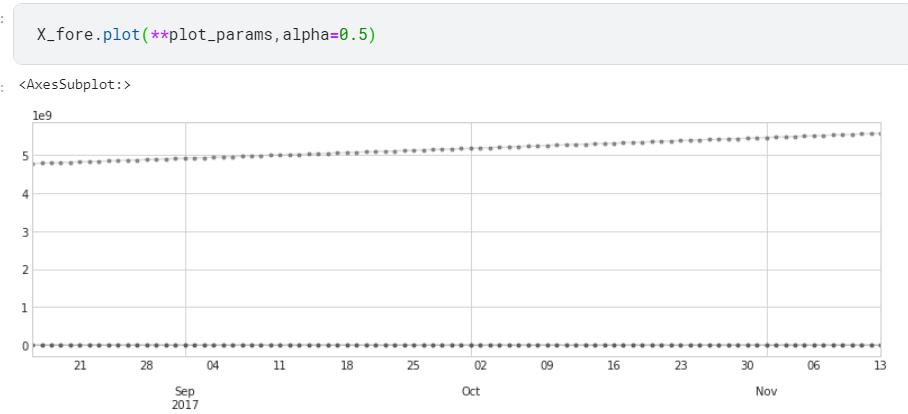``````model = LinearRegression()
model.fit(X,y)
y_pred = pd.Series(model.predict(X),index = X.index)
y_fore = pd.Series(model.predict(X_fore),index = X_fore.index)

ax = y.plot(**plot_params,alpha=0.5,title="Average Sales",ylabel="items sole")
ax = y_pred.plot(ax=ax,linewidth=3,label="Trend",color = "CO")
ax = y_fore.plot(ax=ax,linewidth=3,label="Trend Forecast",color = "C3")
ax.legend();
``````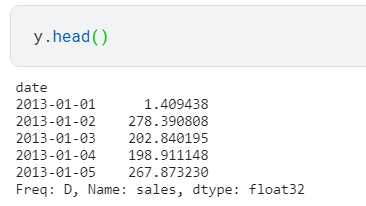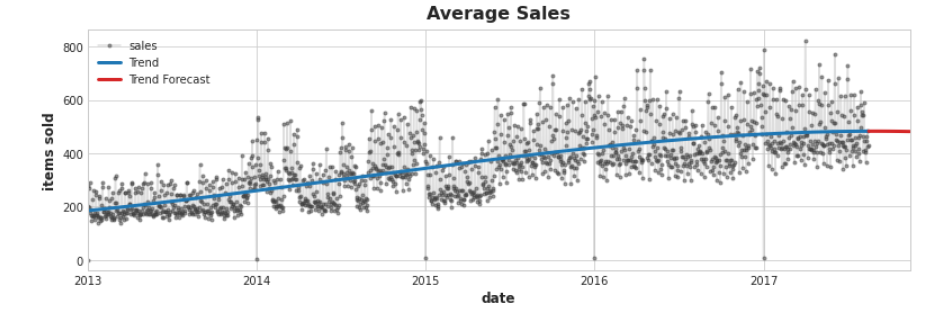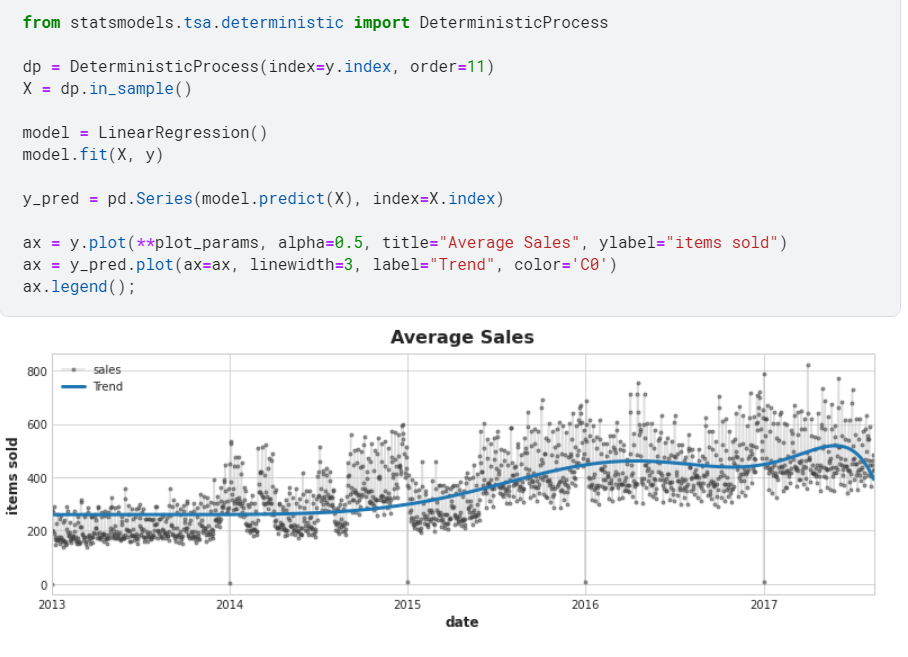4.了解使用高阶多项式进行预测的风险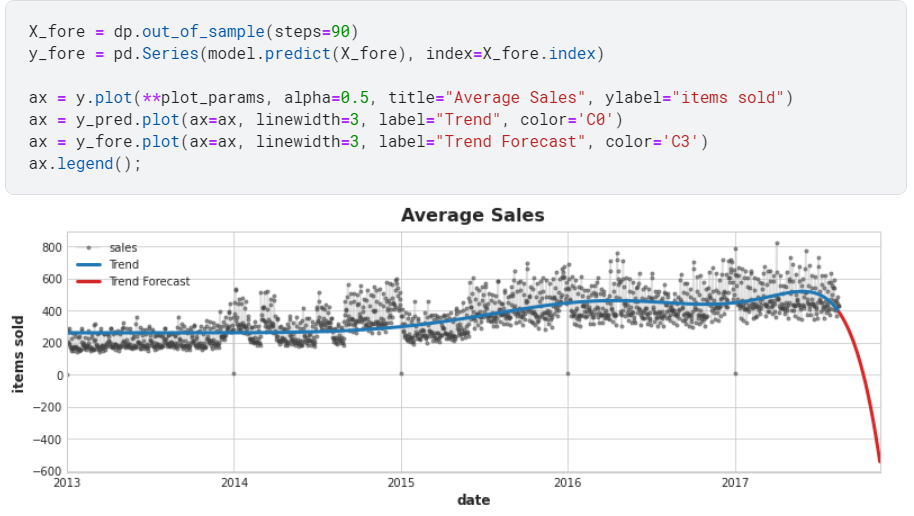5,（可选）用样条拟合趋势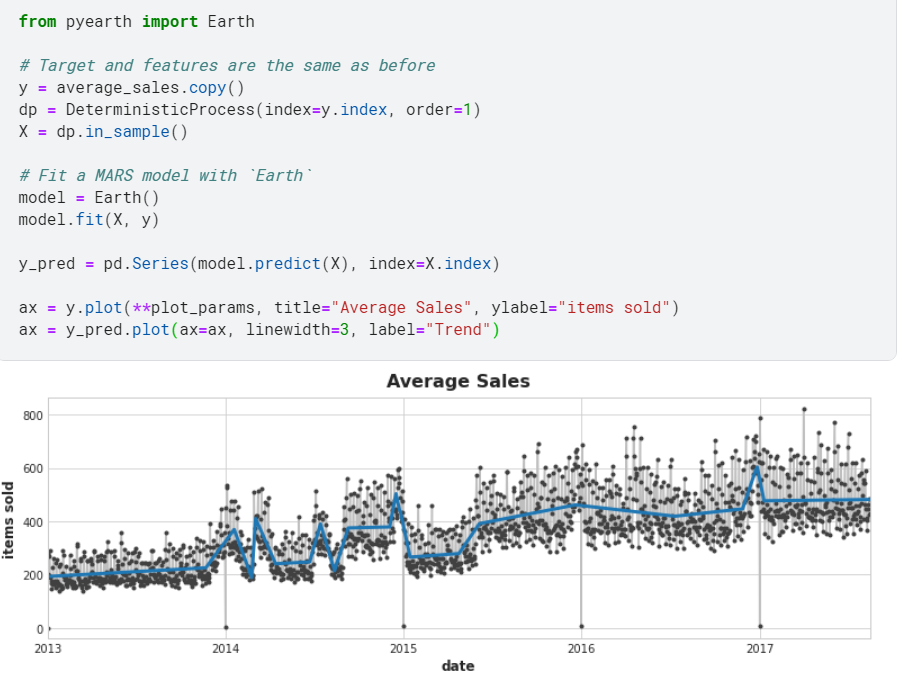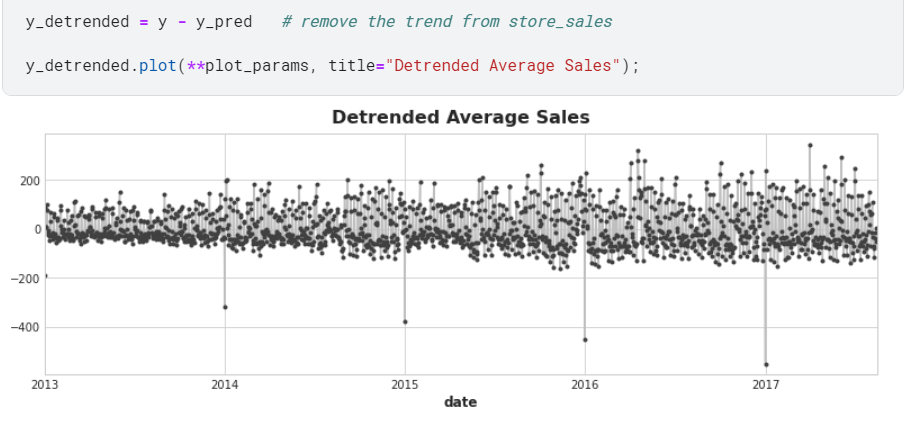THE END